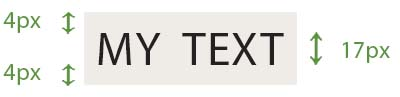# CSS line-height

Jan. 5, 2018 4371The line-height property specifies the height of an inline element. Basically, this property is used to change the space between two lines of text. It is also a component of the font shorthand property.

CSS

line-height: value;


To understand how this property increases or decreases spacing between lines of text, consider an example. Suppose you have a paragraph for which the value of font-size is 17px. Then if you give the line-height value as 25px, then 25px would be the height of the line box which contains the line of text. In that case, the text would cover 17px of the height of the line box and the rest of the space (8px) would be divided equally at the top and bottom of the line of text. Thus, there would be 4px space each at the top and the bottom of the line of text.This works for all the lines of text of the element to which this property is given.

## Values

normal : It sets the default line height which depeneds on the user agent and the font-family.

<number> : This is the number that will be multiplied with the font size to give the line height. For example, the value 1 specifies line height as one times the size of text and the value 2 specifies line height as two times the size of text. Negative values are not allowed.

<length> : It is used to calculate the line box height. It can be given in px, pt, em, rem, and other units. Negative values are not allowed.

<percentage> : It is used to calculate the line height by multiplying this percentage value with the font size, For example, the value 50% specifies line height as 50% the height of the text.

inherit : Inherits the value from parent element.

initial : Sets the initial value for the property.

It is recommended to use a number value listed above for assigning line height to an element. This number is unitless and can even be a decimal number. This is because a child element automatically inherits the line height value from its parent and this can result in inheriting an arbitrary value. So, a giving a number value to the child element can prevent such unexpected results.

## Examples

Consider the following example.

HTML

<p>
This is the first line of first paragraph<br>
This is the second line<br>
This is the third line
</p>

<p id="lheight1">
This is the first line of second paragraph<br>
This is the second line<br>
This is the third line
</p>

<p id="lheight2">
This is the first line of third paragraph<br>
This is the second line<br>
This is the third line
</p>


CSS

body {
font-size: 17px;
}

#lheight1 {
line-height: 1;
}

#lheight2 {
line-height:2;
}


See the Pen CSS Line Height by Aakhya Singh (@aakhya) on CodePen.

Here, the value for the default line height is something around 1.2. This means that the height of the line box is 1.2 times the height of the text. Thus, the space above and below the line of text is .1 times the size of the text.

On setting the value of the line-height property as 1, the heights of the line box and the text became equal, thus leaving no space above or below the text. Similarly, these spaces keep on increasing on increasing the line height value.

Have a look at another example.

HTML

<p>
This is the first line of first paragraph<br>
This is the second line<br>
This is the third line
</p>

<p id="lheight1">
This is the first line of second paragraph<br>
This is the second line<br>
This is the third line
</p>

<p id="lheight2">
This is the first line of third paragraph<br>
This is the second line<br>
This is the third line
</p>

<p id="lheight3">
This is the first line of forth paragraph<br>
This is the second line<br>
This is the third line
</p>


CSS

body {
font-size: 17px;
}

#lheight1 {
line-height: 5px;
}

#lheight2 {
line-height: 17px;
}

#lheight3 {
line-height: 25px;
}


See the Pen CSS Line Height by Aakhya Singh (@aakhya) on CodePen.

In the following example, the line height is set as a percentage of the height of the text.

HTML

<p>
This is the first line of first paragraph<br>
This is the second line<br>
This is the third line
</p>

<p id="lheight1">
This is the first line of second paragraph<br>
This is the second line<br>
This is the third line
</p>

<p id="lheight2">
This is the first line of third paragraph<br>
This is the second line<br>
This is the third line
</p>

<p id="lheight3">
This is the first line of forth paragraph<br>
This is the second line<br>
This is the third line
</p>


CSS

body {
font-size: 17px;
}

#lheight1 {
line-height: 70%;
}

#lheight2 {
line-height:100%;
}

#lheight3 {
line-height:200%;
}


See the Pen CSS Line Height by Aakhya Singh (@aakhya) on CodePen.

## Browser Support

This property is supported by all the major browsers.

Liked the post?
Inquisitive and passionate Front-end Developer and Web Designer and Co-Founder of CodesDope.
0 COMMENT